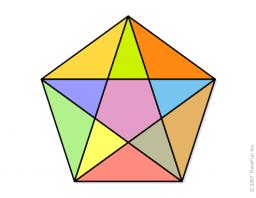# Triangle 2668

The triangle ABC has side lengths a = 14 cm, b = 20 cm, c = 7.5 cm. Find the sizes of the angles and the area of this triangle.

S =  37.3078 cm2
α =  29.8306 °
β =  134.7142 °
γ =  15.4552 °

### Step-by-step explanation:

Try calculation via our triangle calculator.Did you find an error or inaccuracy? Feel free to write us. Thank you!

Tips for related online calculators
Cosine rule uses trigonometric SAS triangle calculator.